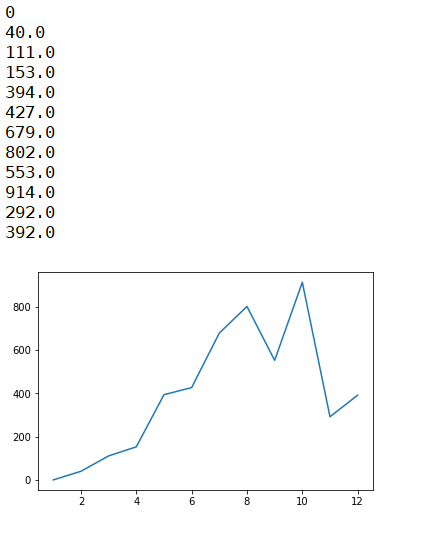# Python program for double biased dice simulation

Double biased dice simulation in Python: Here, we are going to learn how to simulate occurrence of the sum of the faces of two dice [i.e. dice(A) - 1, 2, 3, 4, 4, 4, 5, 6, 6, 6 + dice(B) - 1, 2, 3, 4, 4, 4, 5, 6, 6, 6]?
Submitted by Anuj Singh, on August 01, 2019

Here, we will be simulating the occurrence of the sum of the faces of two dice [i.e. dice(A) - 1, 2, 3, 4, 4, 4, 5, 6, 6, 6 + dice(B) - 1, 2, 3, 4, 4, 4, 5, 6, 6, 6]. Simply we are going to use an inbuilt library called as random to call a random value from given set and thereby we can stimulate the occurrence value by storing the occurrence in the list ls of length 12 representing each face of the dice as ls represents the occurrence of face 5.

```    ls   - dice(1)
ls   - dice(2)
ls   - dice(3)
ls   - dice(4)
ls   - dice(5)
ls   - dice(6)
ls   - dice(7)
ls   - dice(8)
ls   - dice(9)
ls   - dice(10)
ls  - dice(11)
ls  - dice(12)
```

Then using the library pylab, we can plot the value of each occurrence and can stimulate it.

The deviation is clear that each of the faces have an equal almost equal probability of occurrence.

The probability of the 10 is maximum and hence it can be verified by the simulation we have done over more than 2004 times.

The answer for maximum occurrence 10 is as follows:

The occurrence of 4 and 6 is maximum. And hence the probability of 10 and 12 is almost equal but after considering 5:

```    6+4 = 10
4+6 = 10
5+5 = 10
```

Firing of 10 is the most probable and hence we have our peak of the graph at 10.

```    For second peak:
4+4 = 8
4+4 = 8
5+3 = 8
3+5 = 8
6+2 = 8
2+6 = 8
```

And hence the second peak is at 8.

Program:

```import random
import pylab as py

def roll():
return random.choice([1,2,3,4,4,4,5,6,6,6])

ls = [0,0,0,0,0,0,0,0,0,0,0,0]
chance = [104, 203, 302, 401, 505, 646, 756, 855, 985]
for n in chance:
for k in range(n):
scr = roll() + roll()
ls[scr-1] = ls[scr-1] + 4/4

py.figure()
py.plot([1,2,3,4,5,6,7,8,9,10,11,12], ls)

for el in ls:
print(el)
```

OutputPreparation